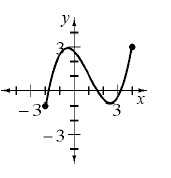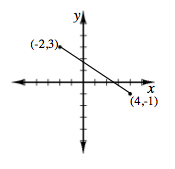### Home > A2C > Chapter 1 > Lesson 1.1.3 > Problem1-35

1-35.

Examine $g(x)$ graphed below.1. Which $x$-values have points on the graph? That is, describe the domain of $g(x)$.

The domain includes $−2, 4$ and all the numbers between. $−2 ≤ x ≤ 4$

2. What are the possible outputs for $g(x)$? That is, what is the range?

The domain includes $−1, 3$, and all the numbers between. $−1 ≤ y ≤ 3$

3. Ricky thinks the range of $g(x)$ is: $−1,0,1,2,\text{ and }3$. Is he correct? Why or why not?

Is there a point on the graph for $x = 2.5$? or for $x = −0.33$?

He is not correct since he is missing all the values between his numbers.
The curve is continuous, so the description needs to include all numbers, not just integers.

4. Draw a graph for another function with the same domain and range as $g(x)$.

There are many possibilities. Here is one example.